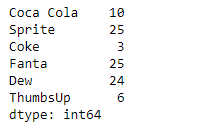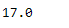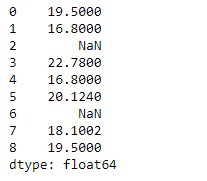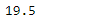# Pandas Series.median()

## Python | Pandas Series.median()

Pandas series is a One-dimensional ndarray with axis labels. The labels need not be unique but must be a hashable type. The object supports both integer- and label-based indexing and provides a host of methods for performing operations involving the index.

Pandas` Series.median()` function return the median of the underlying data in the given Series object.

Syntax: Series.median(axis=None, skipna=None, level=None, numeric_only=None, **kwargs)

Parameter :
axis : Axis for the function to be applied on.
skipna : Exclude NA/null values when computing the result.
level : If the axis is a MultiIndex (hierarchical), count along a particular level, collapsing into a scalar.
numeric_only : Include only float, int, boolean columns
**kwargs : Additional keyword arguments to be passed to the function.

Returns : median : scalar or Series (if level specified)

Example #1: Use `Series.median()` function to find the median of the underlying data in the given series object.

 `# importing pandas as pd` `import` `pandas as pd` ` ` `# Creating the Series` `sr ``=` `pd.Series([``10``, ``25``, ``3``, ``25``, ``24``, ``6``])` ` ` `# Create the Index` `index_ ``=` `[``'Coca Cola'``, ``'Sprite'``, ``'Coke'``, ``'Fanta'``, ``'Dew'``, ``'ThumbsUp'``]` ` ` `# set the index` `sr.index ``=` `index_` ` ` `# Print the series` `print``(sr)`

Output :Now we will use `Series.median()` function to find the median of the given series object.

 `# return the median` `result ``=` `sr.median()` ` ` `# Print the result` `print``(result)`

Output :As we can see in the output, the `Series.median()` function has successfully returned the median of the given series object.

Example #2: Use `Series.median()` function to find the median of the underlying data in the given series object. The given series object contains some missing values.

 `# importing pandas as pd` `import` `pandas as pd` ` ` `# Creating the Series` `sr ``=` `pd.Series([``19.5``, ``16.8``, ``None``, ``22.78``, ``16.8``, ``20.124``, ``None``, ``18.1002``, ``19.5``])` ` ` `# Print the series` `print``(sr)`

Output :Now we will use `Series.median()` function to find the median of the given series object. we are going to skip the missing values while calculating the median in the given series object.

 `# return the median` `result ``=` `sr.median(skipna ``=` `True``)` ` ` `# Print the result` `print``(result)`

Output :As we can see in the output, the `Series.median()` function has successfully returned the median of the given series object.

Last Updated on October 24, 2021 by admin

## Pandas dataframe.get_valuePandas dataframe.get_value

The get_value() method in the Pandas library is used to retrieve a scalar value from

## Concatenate Pandas DataFrames Without DuplicatesConcatenate Pandas DataFrames Without Duplicates

Concatenate Pandas DataFrames Without Duplicates In this article, we are going to concatenate two dataframes

## Create a correlation Matrix using PythonCreate a correlation Matrix using Python

Create a correlation Matrix using Python A correlation matrix is a table containing correlation coefficients

## Convert Floats to Integers in a Pandas DataFrameConvert Floats to Integers in a Pandas DataFrame

Convert Floats to Integers in a Pandas DataFrame Let us see how to convert float

## Creating Pandas dataframe using list of listsCreating Pandas dataframe using list of lists

Pandas DataFrame is a 2-dimensional labeled data structure with columns of potentially different types. It

## Pandas Series.ravel()Pandas Series.ravel()

Python | Pandas Series.ravel() Pandas series is a One-dimensional ndarray with axis labels. The labels

## Python – Pandas Dataframe.sample()Python – Pandas Dataframe.sample()

Python Pandas Dataframe.sample()   Pandas sample() is used to generate a sample random row or